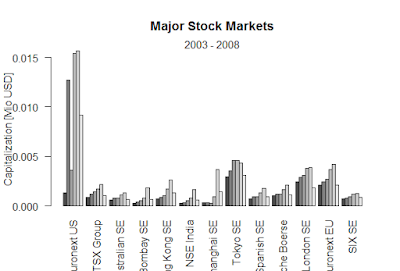Showing posts with label Implied volatility. Show all posts
Showing posts with label Implied volatility. Show all posts

## Implied volatility

Implied volatility is the focus of interest both in volatility trading and in risk management. Implied volatility may be used as an indicator of market expectations over the remaining lifetime of the option. The analysis of volatility in the financial market has become of the first rank issue in modern financial theory and practice whenever in a risk management for polio hedging for option pricing we need to have a person is the notion of the market expectations of volatility.
Implied volatility is well known that the volatility employed by observing market prices tattoo that is far different from the flat constant one used in the black Scholes formula.
We focus first on the static and visual investigation of implied volatility then we concentrate on a dynamic analysis with two variants of principal components and interpret the result in the context of risk management.
Traders directly trade with Vega it is the sensitivity of their portfolio with respect to volatility changes. Rekha trader market professionals use Delta gamma new hatching stage de which are insane to changes in the underlying and to time decay. Vega is the first derivative of the black Scholes formula with respect to the volatility parameter and can be interpreted as the sensitivity of the option value with respect to change in implied volatility. We are using principal components analysis to determine the maximum loss of options for folio bearing Vega exposure.

### Black-Scholes formula-R

Black-Scholes formula-R > BlackScholes <- function(TypeFlag = c("c", "p"), S, X, Time, r, b, sigma) { TypeFla...